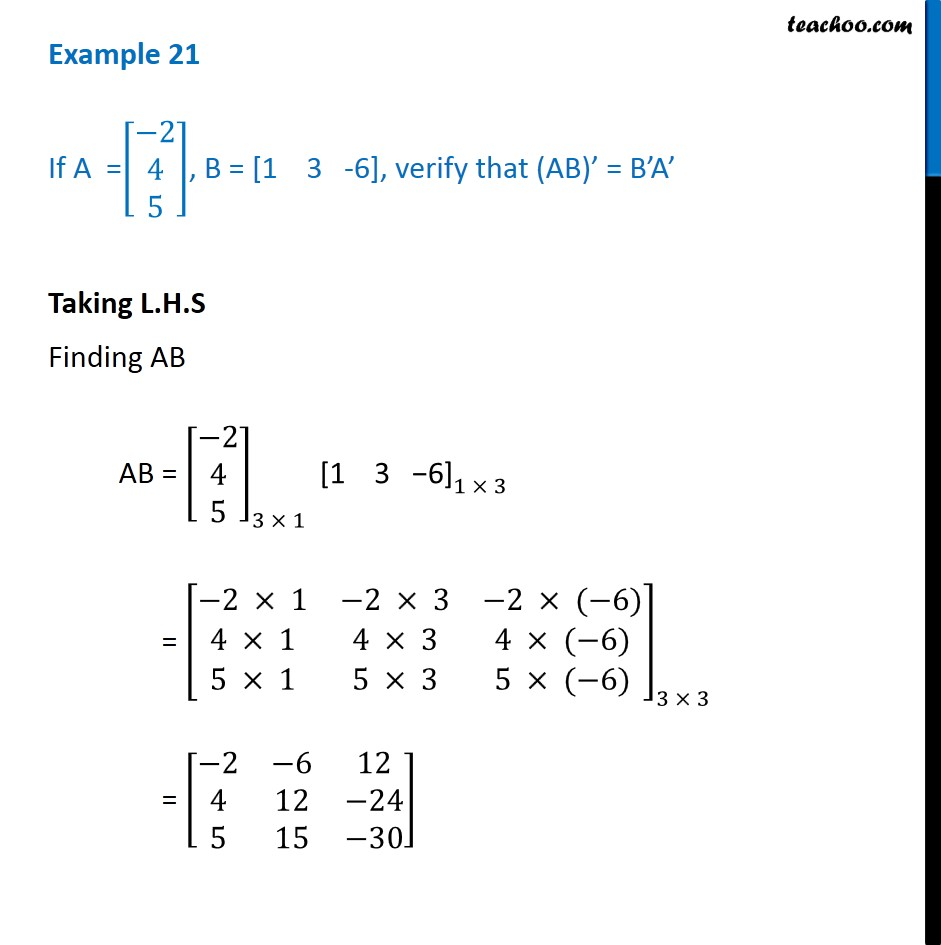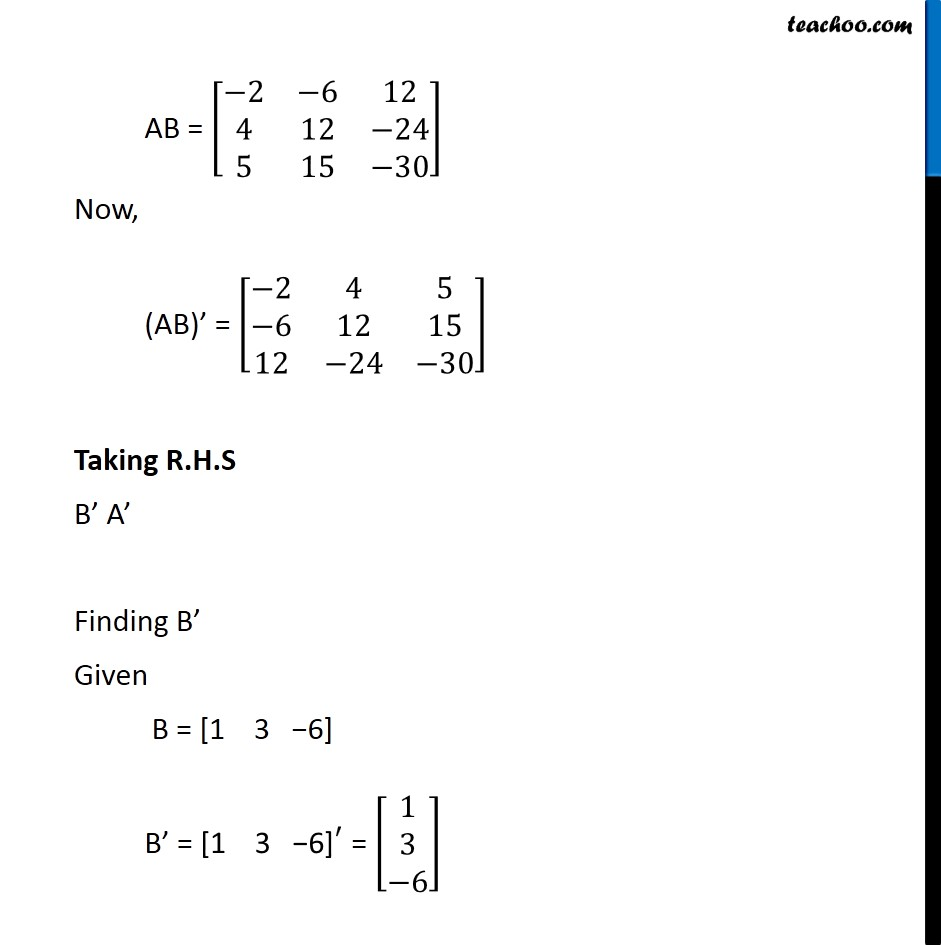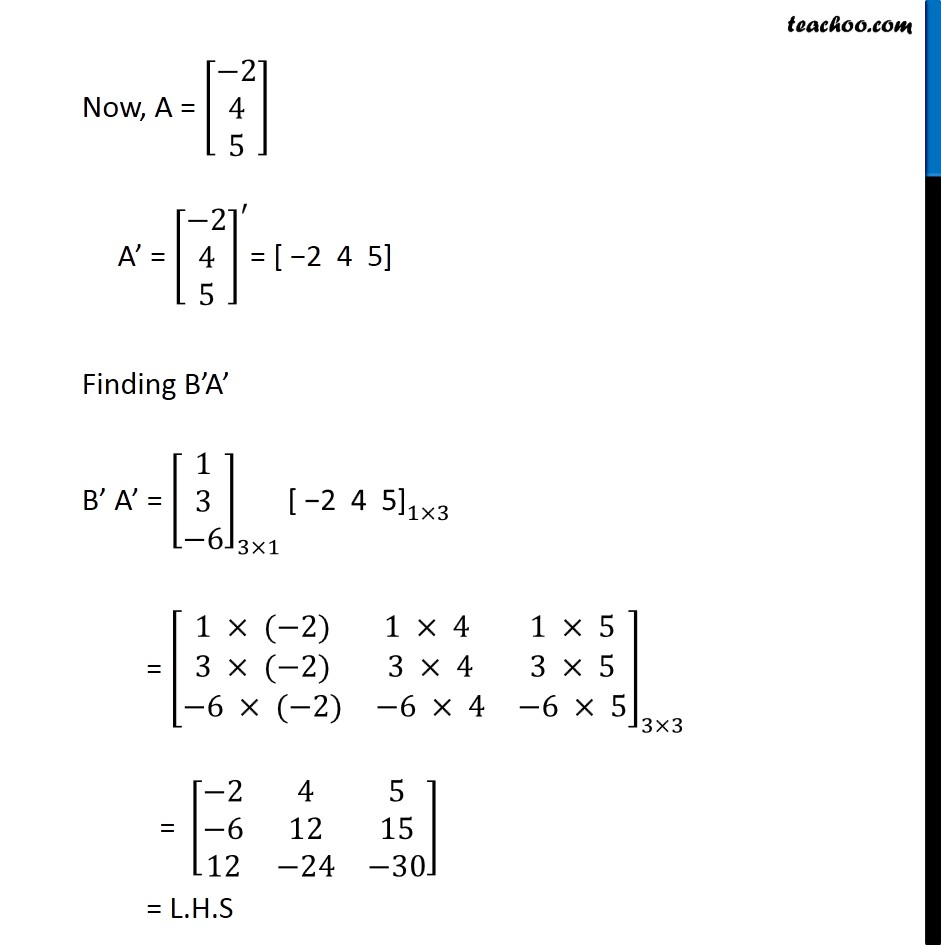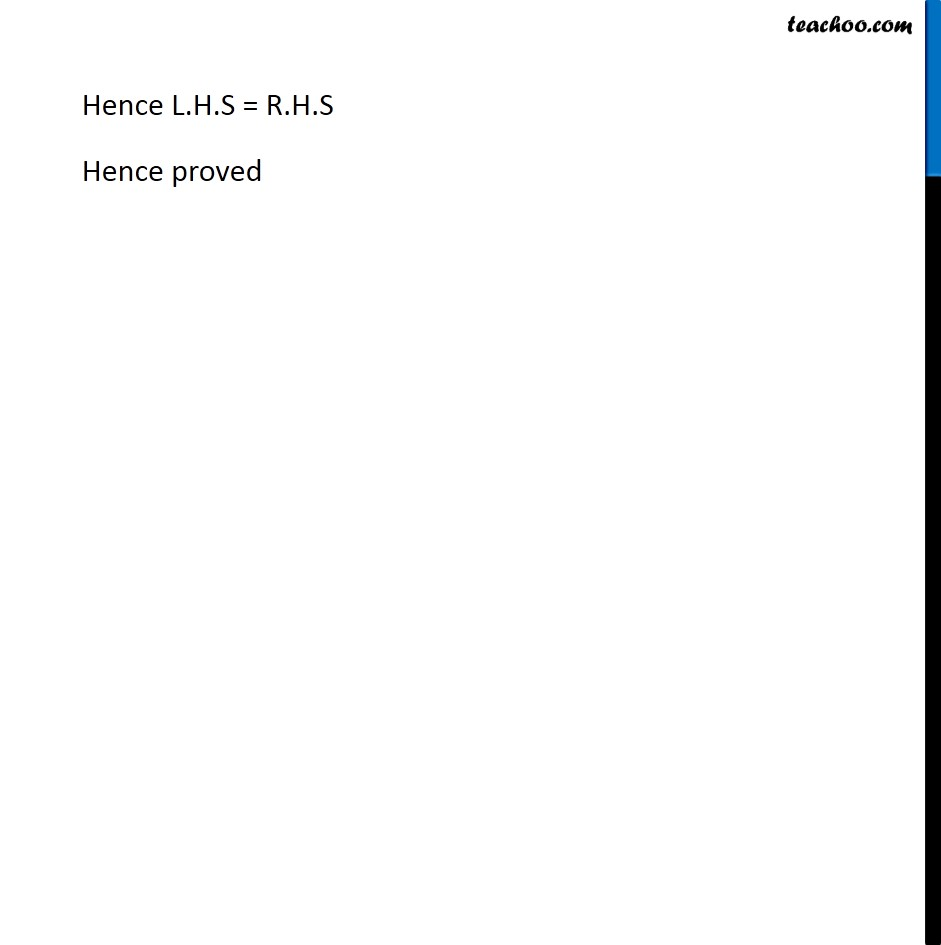Transpose of a matrix

Chapter 3 Class 12 Matrices (Term 1)
Concept wise### Transcript

Example 21 If A =[■8(−2@4@5)], B = [1 3 -6], verify that (AB)’ = B’A’ Taking L.H.S Finding AB AB = [■8(−2@4@5)]_(3 × 1) 〖"[1 3 −6]" 〗_(1 × 3) = [■8(−2 × 1&−2 × 3&−2 × (−6)@4 × 1&4 × 3&4 × (−6)@5 × 1&5 × 3&5 × (−6))]_(3 × 3) = [■8(−2&−6&12@4&12&−24@5&15&−30)] AB = [■8(−2&−6&12@4&12&−24@5&15&−30)] Now, (AB)’ = [■8(−2&4&5@−6&12&15@12&−24&−30)] Taking R.H.S B’ A’ Finding B’ Given B = "[1 3 −6]" B’ = 〖"[1 3 −6]" 〗^′ = [■8(1@3@−6)] Now, A = [■8(−2@4@5)] A’ = [■8(−2@4@5)]^′= [ −2 4 5] Finding B’A’ B’ A’ = [■8(1@3@−6)]_(3×1) 〖"[ −2 4 5]" 〗_(1×3) = [■8(1 × (−2)&1 × 4 &1 × 5@3 × (−2)&3 × 4&3 × 5@−6 × (−2)&−6 × 4&−6 × 5)]_(3×3) = [■8(−2&4&5@−6&12&15@12&−24&−30)] = L.H.S Hence L.H.S = R.H.S Hence proved